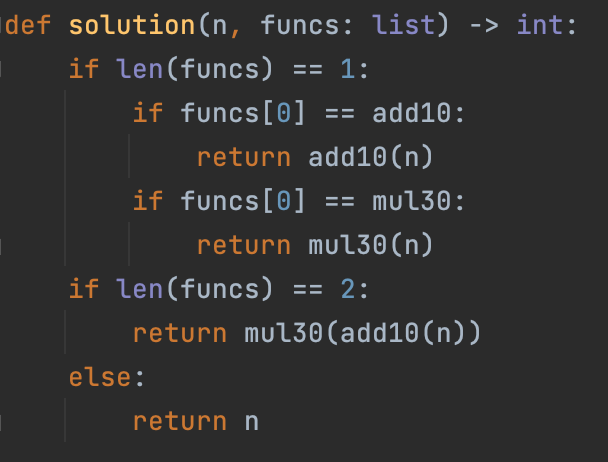# 【每日一题20220610】链式计算‍ 给定一个初始数字和一个函数对象列表，我们的任务就是，将初始值依次传入函数中，后续的函数输入正是前面函数的输出。请编写一个函数，计算出最终的结果值。

【示例】

``````def add10(x): return x + 10
def mul30(x): return x * 30
``````

``````def solution(n, funcs: list)-> int:

assert solution(50, [add10, mul30]) == 1800
assert solution(50, [mul30]) == 1500
assert solution(42, []) == 42
``````
``````def add10(x): return x + 10
def mul30(x): return x * 30

def solution(n, funcs: list) -> int:
if (l := len(funcs)) > 0:
return funcs[l-1](solution(n, funcs[:l-1]))
else:
return n

assert solution(50, [add10, mul30]) == 1800
assert solution(50, [mul30]) == 1500
assert solution(42, []) == 42
``````
1 Like
``````def solution(n, funcs: list)-> int:
if funcs is None:
return n
for i in funcs:
n = i(n)
return n

assert solution(50, [add10, mul30]) == 1800
assert solution(50, [mul30]) == 1500
assert solution(42, []) == 42
``````
1 Like
``````def solution(n, funcs: list) -> int:
if len(funcs):
for fun in funcs:
n = fun(n)
return n
return n
``````

assert solution(50, [add10, mul30]) == 1800
assert solution(50, [mul30]) == 1500
assert solution(42, ) == 42

1 Like``````def solution(n, funcs: list)-> int:
for func in funcs:
n=func (n)
return n

``````
``````def solution(n, funcs: list)-> int:
if funcs==[]:
return n
for i in funcs:
n=i(n)
return n
``````